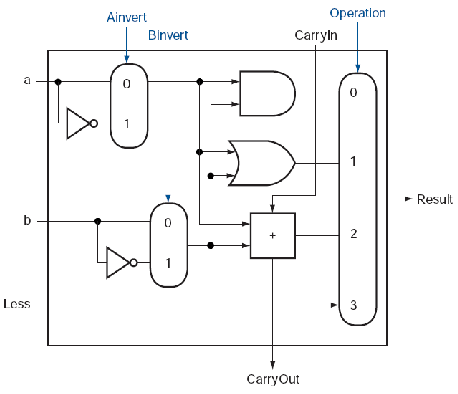Homework V

Tom Kelliher, CS 240

50 points, due April. 9

For this assignment, you will be implementing in VHDL and simulating an eight-bit version of the simplified ALU used in Patterson & Hennessy's Computer Organization & Design textbook. Use the following for the top-level entity I/O structure of your ALU:

```entity alu is
Port ( a        : in   STD_LOGIC_VECTOR (7 downto 0);
b        : in   STD_LOGIC_VECTOR (7 downto 0);
op       : in   STD_LOGIC_VECTOR (2 downto 0);
result   : out  STD_LOGIC_VECTOR (7 downto 0);
overflow : out  STD_LOGIC
);
end alu;
```
The functions to be computed by the ALU are defined by:
```if (op == 0)
result = a AND b;
else if (op == 1)
result = a OR b;
else if (op == 2)
result = a NAND b;
else if (op == 3)
result = a NOR b;
else if (op == 4)
{
result = a + b;
if (overflow has occurred)
overflow = 1;
else
overflow = 0;
}
else if (op == 5)
{
result = a - b;
if (overflow has occurred)
overflow = 1;
else
overflow = 0;
}
else if (op == 6)   // set on less than AKA slt
if (a < b)
result = 1;
else
result = 0;
```
For those ops for which `overflow` is not defined, you may assume that it is a don't care. (Don't read too much into this -- it simply means that you only need be concerned with overflow's value for the addition and subtraction operations.) You may assume that op's value will never be 7.

You will find this schematic useful as a starting point for a one-bit ALU component which you'll instantiate multiple times to implement the eight-bit ALU:You'll need to make a few changes when implementing this schematic:

1. The slt operation requires access to the sign bit. This operation should perform correctly for all values of a and b, even if overflow occurs. (Remember, overflow indicates that the sign bit is wrong.)

2. The simplest way of recognizing when overflow has occurred is by comparing the carryouts from the two most significant bits. If they differ, overflow has occurred; otherwise, there is no overflow.

3. Recall that VHDL has an addition operator. Hence, there is no need for you to implement a one-bit full adder.

Turn in a listing of your VHDL code, your simulation waveform(s), and a brief rationale addressing why I should be convinced that your simulation demonstrates the correctness of your VHDL design. You will be graded on the correctness of your VHDL as well as on the simulation test set you have chosen.

Thomas P. Kelliher 2010-03-31
Tom Kelliher